### Voice Recognition Model¶

Hi all! When I first entered machine learning there were a few use cases that I thought were absolutely amazing. I had a strong background in statistics (a bachelors in physics can do that to you), but many of the models I saw in the field seemed more like black magic than processes generated from mathematical principles.

So I did what any good scientist might do, I set out to build simple proofs of concepts. I made chat bots, reinforcement learners, computer vision models. I tried methodologies that were decades old and ones that were brand new. I'm still amazed at how much of what I learned messing around with these toy model came back to help me in actual production model builds later in my career.

To this end, I wanted to publish some of the more fun projects that I toyed around with. I've tried to clean up some of these older projects (I'm not the best coder now but I was much worse back when I wrote these) so please forgive any residual sloppiness.

So, what are we learning about today? Voice recognition! While audio recordings are jsut signals and signal processing/modeling is a very well covered discipline, I've found that a lot of new data scientists struggle to work with sound. However, there are some super cool things that you can do with sound. For example, I've been working with auscultatory (sound) recordings taken of the chest to try to diagnose heart pathologies. You could also create models to try and triangulate exact location of a gun shot in a city based on recordings from multiple speakers placed in the vicinity of the gunshot.

Today we'll do something far less exciting: voice recognition. We won't be exploring the state of the art in voice recognition (which is based in deep learning, is pretty complicated, and requires more data and computing power than you or I probably want to bother with). Instead, I'll show you a simple modeling approach that works decently using Gaussian mixture models.

To get to the final model we'll need to cover a lot of pre-processing material. Here's our outline:

• Preprocessing
• Pre-emphasis
• Removing Silence
• Normalize Energy Density
• Framing
• Windowing
• Fourier-Transform and Power Spectrum
• Filter Banks
• Mel-frequency Cepstral Coefficients (MFCCs)
• Mean Normalization
• Filter Banks vs MFCCs
• Modeling the Human Voice

If you are new to signal processing and/or time series analysis, I would recommend reading through the first sections of my time series course before delving into this tutorial. I assume familiarity with concepts like Fourier transforms, stationarity, and basis functions/frequencies.

### Preprocessing¶

We'll start by learning how to augment our audio signals in such a way that we can extract as many interesting and salient features as possible. As you can see below, the raw audio signal is just a one dimensional vector. We'll work to augment the same such that we learn about the frequencies that comprise the signal and the power distribution of those frequencies. These are the features that really make our voices unique.

I will largely draw from this blog for pre-processing with a few changes and exceptions: Speech Processing for Machine Learning: Filter banks, Mel-Frequency Cepstral Coefficients (MFCCs) and What's In-Between

In :
import numpy as np
import numpy
import scipy.io.wavfile
from scipy.fftpack import dct

from sklearn import mixture
from sklearn.externals import joblib
import glob

import matplotlib.pyplot as plt
from matplotlib import cm
import seaborn as sns
import librosa

import IPython.display as ipd
import librosa.display

import scipy.stats as st

In :
# I really like librosa for audio processing in python
# as well as to just display samples
# however, librosa handles much of the nitty gitty for us
# so I'll switch to scipy to teach the preprocessing concepts
# and then back to librosa when we get to the actual model build
# it's good to get a taste of the nitty gritty to make sure
# you know how the sausage is made!

print("Audio Signal Vector: \n{}".format(x))

plt.figure(figsize=(12, 4))
librosa.display.waveplot(x, sr=sr)
plt.title('Signal')
plt.ylabel('Amplitude')
plt.xlabel('Time (Sec)')
plt.show()

ipd.Audio(x, rate=sr)

Audio Signal Vector:
[-1.2653547e-06  1.4176046e-06 -1.1516612e-06 ... -1.8368870e-03
-2.2029171e-03 -2.1080920e-03]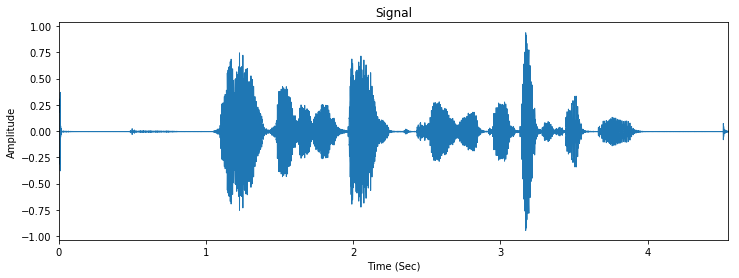Out:

We'll start with a few basic transforms in the time domain. Eventually we'll want to start working with our signal in the frequency domain which has much more rich features for our model to work with. The frequencies of our voices can tell us a lot such as gender, age, etc.

Our first transform is a pre-emphasis filter that amplifies higher frequencies in our signal. We usually do this for a few different reasons:

• High frequencies often have lower power magnitudes than lower frequencies and our amplification can add some balance to the signal. This can also helps prevent loss of information in the higher frequency spectrum in later transformations.
• This can help avoid numerical issues with Fourier transforms.
• Can act to improve signal-to-noise ratio.

Honestly, we often skip the pre-emphasis filter these days as signal processing methods have improved drastically as more computing power has become available to modelers. The benefits of pre-emphasis filters become negligible when one applies transforms like mean normalization (which I address later).

However, it's a nice method to know about in case you want a quick transform and choose not to use mean normalization later in the preprocessing pipeline.

The formula for our filter is as follows:

$y(t) = x(t) - \alpha x(t-1)$

I show before and after plots of our signal below.

In :
sample_rate, signal = scipy.io.wavfile.read('James_train.wav')
i = 5.5
signal = signal[0:int(i * sample_rate)]  # Keep the first i seconds

In :
Time=np.linspace(0, len(signal)/sample_rate, num=len(signal))

plt.figure(figsize=(20,5))
plt.plot(Time,signal[:,0])
plt.title('Signal')
plt.ylabel('Amplitude')
plt.xlabel('Time (Sec)')
plt.show()

pre_emphasis = 0.97
emphasized_signal = numpy.append(signal, signal[1:] - pre_emphasis * signal[:-1])

Time=np.linspace(0, len(emphasized_signal)/sample_rate, num=len(emphasized_signal))

plt.figure(figsize=(20,5))
plt.plot(Time,emphasized_signal)
plt.title('Emphasized Signal')
plt.ylabel('Amplitude')
plt.xlabel('Time (Sec)')
plt.show()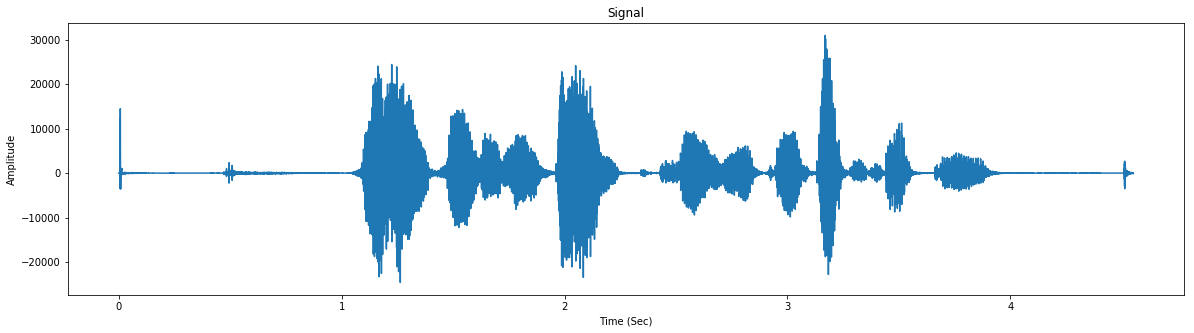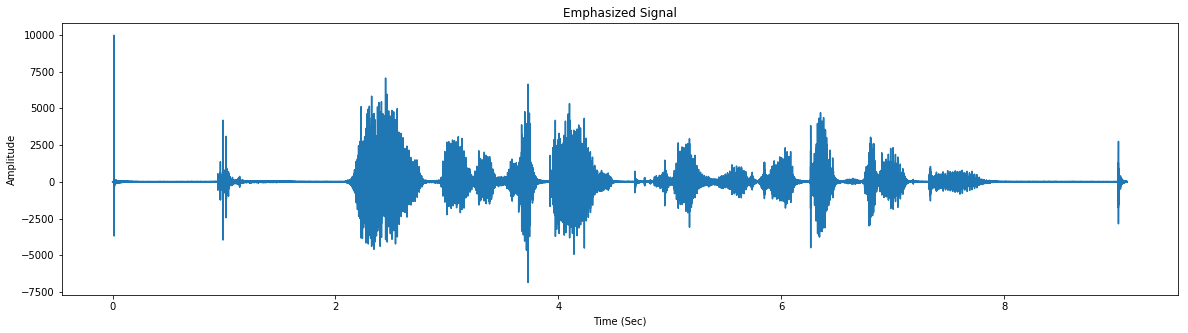Okay, next we want to discard the useless parts of our sample. Namely, we'll remove the silent parts. Dealing with silence is difficult. We could use the silence to try to model certain speech patterns (maybe the speaker hesitates or stutters more than most people), but often enough the amount and length of silence in our speech depends highly on context. We want our model to identify a voice independent of the context in which the voice sample was taken (i.e. shouting, whispering, fast, slow).

Removing silence can be tricky! Below I implement a simple thresh holding filter that removes any vector value below a threshold. Librosa also has a function to do this which I sue to compare to my filter.

I will note, some modelers prefer to skip the removing silence and energy normalization steps. I won't weigh in on this debate, but I encourage to try building models with and without these steps and choose a method for yourself.

In :
plt.figure(figsize=(20,5))
plt.plot(Time,emphasized_signal)
plt.title('Emphasized Signal')
plt.ylabel('Amplitude')
plt.xlabel('Time (Sec)')
plt.show()

s = emphasized_signal[ numpy.absolute(emphasized_signal) > 30]

plt.figure(figsize=(20,5))
plt.plot(s)
plt.title('Emphasized Signal without Silence')
plt.ylabel('Amplitude')
plt.show()

# remove silence
y = librosa.effects.split(emphasized_signal,top_db=30)
l = []
for i in y:
l.append( emphasized_signal[i:i] )
emphasized_signal = np.concatenate(l,axis=0)

Time=np.linspace(0, len(emphasized_signal)/sample_rate, num=len(emphasized_signal))

plt.figure(figsize=(20,5))
plt.plot(Time,emphasized_signal)
plt.title('Emphasized Signal without Silence (Librosa)')
plt.ylabel('Amplitude')
plt.xlabel('Time (Sec)')
plt.show()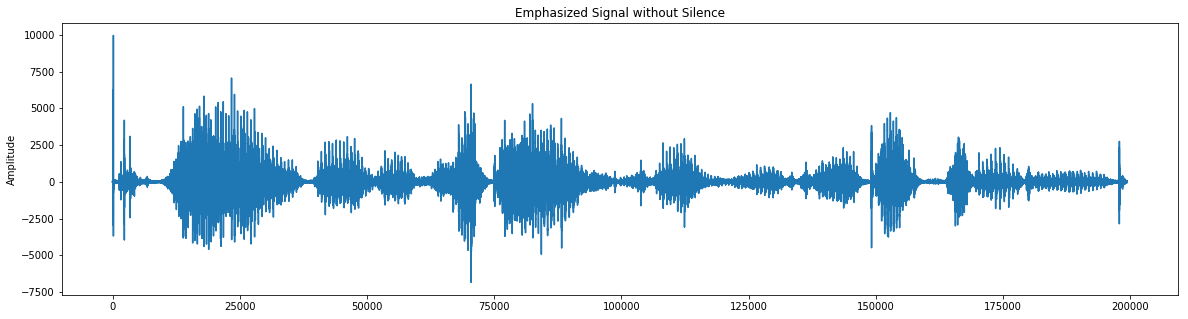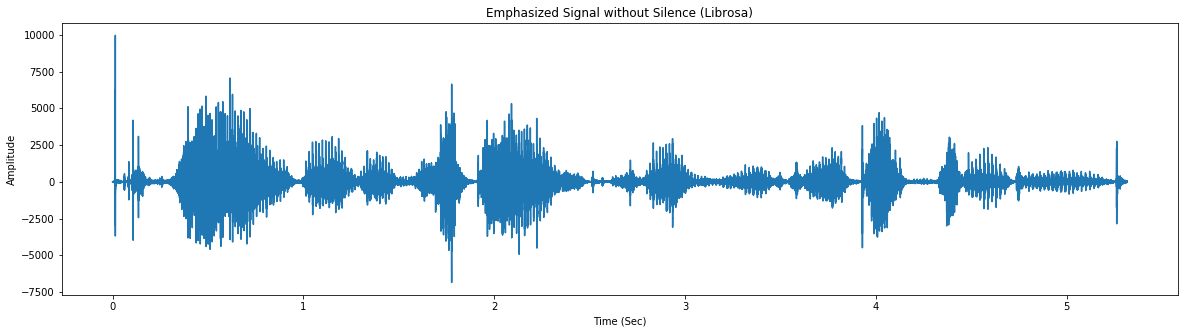Try listening to both filtered examples (mine and librosa's) below. You'll notice that librosa does a MUCH better job of filtering silence. We'll go with librosa's filter, but at least you have an idea of how you could roll your own if you wanted to.

In :
print("My Augmentation")
ipd.Audio(s, rate=2*sample_rate)

My Augmentation

Out: